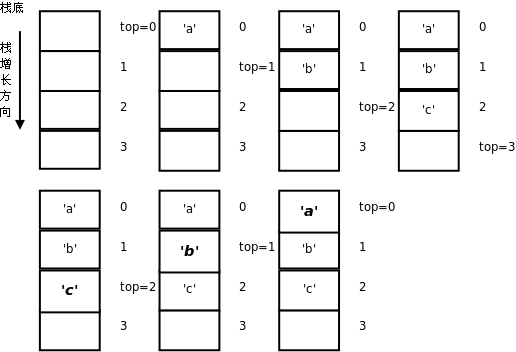## 2. 堆栈

```#include <stdio.h>

char stack;
int top = 0;

void push(char c)
{
stack[top++] = c;
}

char pop(void)
{
return stack[--top];
}

int is_empty(void)
{
}

int main(void)
{
push('a');
push('b');
push('c');

while(!is_empty())
putchar(pop());
putchar('\n');

return 0;
}````main`函数中，入栈的顺序是`'a'``'b'``'c'`，而出栈打印的顺序却是`'c'``'b'``'a'`，最后入栈的`'c'`最早出来，因此堆栈这种数据结构的特点可以概括为LIFO（Last In First Out，后进先出）。我们也可以写一个递归函数做倒序打印，利用函数调用的栈帧实现后进先出：

```#include <stdio.h>
#define LEN 3

char buf[LEN]={'a', 'b', 'c'};

void print_backward(int pos)
{
if(pos == LEN)
return;
print_backward(pos+1);
putchar(buf[pos]);
}

int main(void)
{
print_backward(0);
putchar('\n');

return 0;
}```

```for (i = LEN-1; i >= 0; i--)
putchar(buf[i]);```